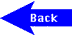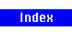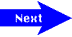# National Aeronautics and Space Administration

## Goddard Space Flight Center## SPACE SQUARED - Level 2

Joseph L. Lagrange was a French mathematician who lived from 1736 to 1813. He made many contributions to the field of mathematics, but the most notable were the calculus of variations and the development of the metric system. Lagrange was also an amateur astronomer. His two fields of interest, mathematics and astronomy, have been combined in this activity.

Joseph Lagrange proved conclusively that the Four Square Theorem was indeed a valid theorem. This theorem states that every positive integer is expressible as a sum of four or fewer square numbers. A square number is attained when a number is multiplied times itself (example: 3 X 3 = 9; 9 is a square number).

Below you will find the distances between selected space objects. Your mission is to take the underlined number and express it as a sum of four or fewer square numbers. There may be more than one right answer. The first one is done for you.

1. Earth to Barnard's Star - 6 lightyears
Solution: 1 + 1 + 4 = 6   (1x1 + 1x1 + 2x2)

2. Earth to Ursa Major - 7 lightyears

3. Sun to Earth (average) - 150 million kilometers

4. Venus to Earth (average) - 42 million kilometers

5. Mercury to Mars (average) - 170 million kilometers

6. Earth to Pleiades Star Cluster - 400 lightyears

7. Uranus to Neptune (average) - 1630 million kilometers

8. Earth to the star Vega - 27 lightyears

9. Earth to M51: Whirlpool Galaxy - 14 million lightyears

10. Center of the Milky Way Galaxy to the Sun - 30,000 lightyearsA service of the High Energy Astrophysics Science Archive Research Center (HEASARC), Dr. Alan Smale (Director), within the Astrophysics Science Division (ASD) at NASA/GSFC